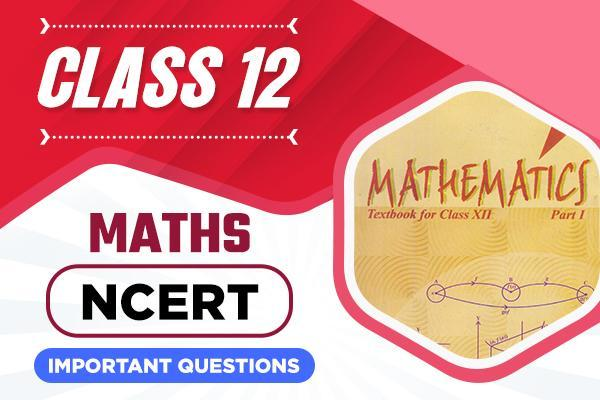Feedback## Important Questions for Class 12 Maths Chapter Wise

Class 12 NCERT important Questions and Examples.

Language: English

Class 12 Mathematics chapter wise important questions and examples for May/June 2021 exams by CBSE experts. This includes important NCERT questions and examples of chapiter 1 relation and functions, chapter 2 inverse trigonometry, chapter 3 matrices chapter 4 determinants, chapter 5 differentiation, chapter 6 applications of derivatives (AOD), chapter 7 integration, chapter 9 application of integration, chapter 10 differential equations, chapter 11 vectors and three dimensional, chapter 12 linear programming, chapter 13 probability.

Class 12 CBSE sample papers with marking scheme and suggested answers are also available for May/June 2021.

Still any doubt?